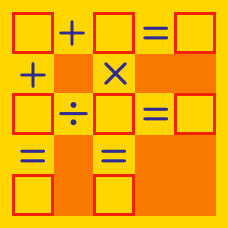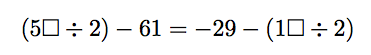Logic

# Arithmetic Puzzles - Fill in the Blanks

If $$2\square + 35 = 58,$$ what is $$\square?$$

This is an arithmetic puzzle, where $$1 \square$$ would represent the 2-digit number 19 if $$\square = 9$$. It does not represent the algebraic expression $$1 \times \square$$.

If $$(1 \square \times 3) +8= 59,$$ what number should be placed in $$\square?$$

This is an arithmetic puzzle, where $$1 \square$$ would represent the 2-digit number 19 if $$\square = 9$$. It does not represent the algebraic expression $$1 \times \square$$.

If $$(5 \square \div 2) -61= -35,$$ what number should be placed in $$\square?$$

This is an arithmetic puzzle, where $$1 \square$$ would represent the 2-digit number 19 if $$\square = 9$$. It does not represent the algebraic expression $$1 \times \square$$.Both boxes contain the same digit. What digit would make the equation true?

This is an arithmetic puzzle, where $$1 \square$$ would represent the 2-digit number 19 if $$\square = 9$$. It does not represent the algebraic expression $$1 \times \square$$.

If $$2 \square \times 4 = 88,$$ what number should be placed in $$\square?$$

This is an arithmetic puzzle, where $$1 \square$$ would represent the 2-digit number 19 if $$\square = 9$$. It does not represent the algebraic expression $$1 \times \square$$.

×